Courses

# Heat Exchanger - 2

## 10 Questions MCQ Test Heat Transfer | Heat Exchanger - 2

Description
This mock test of Heat Exchanger - 2 for Chemical Engineering helps you for every Chemical Engineering entrance exam. This contains 10 Multiple Choice Questions for Chemical Engineering Heat Exchanger - 2 (mcq) to study with solutions a complete question bank. The solved questions answers in this Heat Exchanger - 2 quiz give you a good mix of easy questions and tough questions. Chemical Engineering students definitely take this Heat Exchanger - 2 exercise for a better result in the exam. You can find other Heat Exchanger - 2 extra questions, long questions & short questions for Chemical Engineering on EduRev as well by searching above.
QUESTION: 1

Solution:
QUESTION: 2

Solution:
QUESTION: 3

### The overall heat transfer coefficient for a shell and tube heat exchanger for clean surfaces is Uo = 400 W/m2K. The fouling factor after one year of operation is found to be hdo = 2000 W/m2K. The overall heat transfer coefficient at this time is

Solution: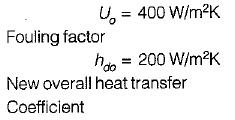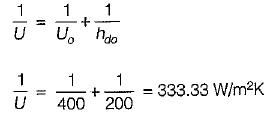QUESTION: 4

A solar assisted air conditioning system, 1 kg/sec of ambient air is to be preheated by the same amount of air leaving the system. A counter-flow heat exchanger having an area of 60 m2 with overall heat transfer coefficient of 25 W/m2K is used for this purpose. Assuming cp for air is 1 kJ/kgK. The effectiveness of the heat exchanger is

Solution: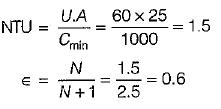QUESTION: 5

Hot air enters a single pass counter flow heat exchanger at 700 K and leaves at 400 K the cooling water enters at 300 K and leaves at 600 K. The LMTD for the heat exchanger is

Solution: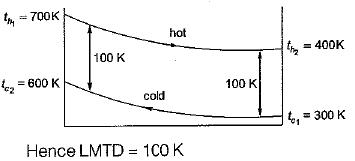QUESTION: 6

For the same inlet and outlet temperatures of hot and cold fluid, the LMTD is

Solution:
QUESTION: 7

Which one of the following graphs, showing temperature distributions of different heat exchangers, pertains steam condenser

Solution:
QUESTION: 8

In which type of heat exchanger is the inlet temperature difference between the hot and cold fluids is maximum

Solution:

for parallel flow heat exchanger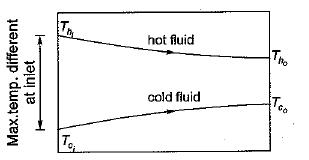QUESTION: 9

In a counter flow heat exchanger, the product of specific heat and mass flow rate is same for the hot and cold fluids. If NTU is equal to 0.5 then the effectiveness of the heat exchanger is

Solution: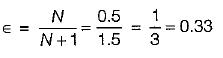QUESTION: 10

When tc1 and tc2 are the temperatures of cold fluid at entry and exit respectively and th1 and th2 are the temperature of hot fluid at entry and exit point and cold fluid has lower heat capacity rate as compared to hot fluid then effectiveness of the heat exchanger is given by

Solution: